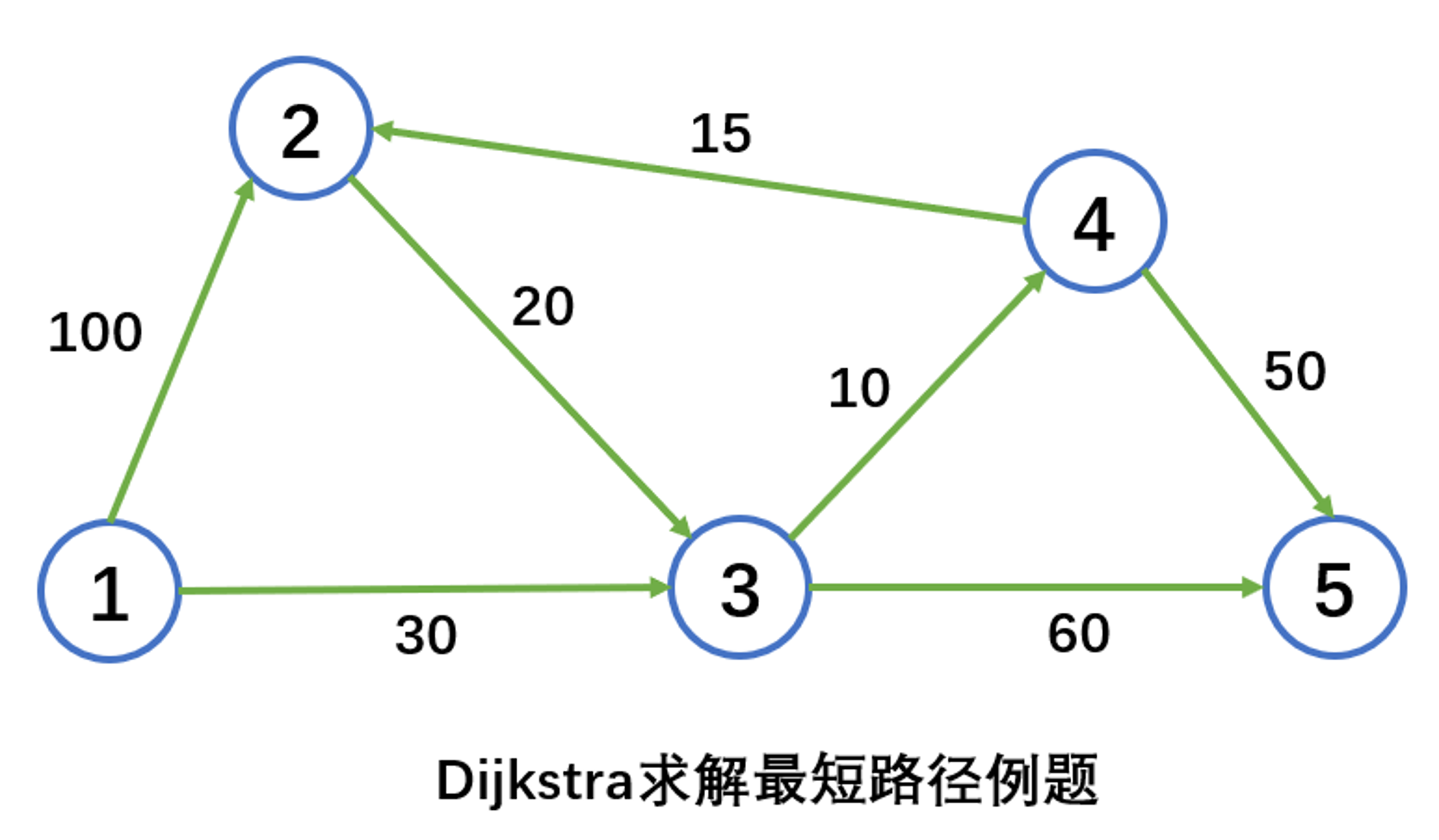墨滴2021/08/29  阅读：107  主题：默认主题

最短路径—迪杰斯特拉算法(Dijkstra)

最短路径算法——迪杰斯特拉算法(Dijkstra)

1迪杰斯特拉算法(Dijkstra)

1.2 步骤

<1>计算节点 有边相连的每一个暂时节点 不是永久的） . 如果节点 已经被另一个节点 连接并表示为 ，并且\$s_i + d_{ij}

<2> 如果所有节点都是永久的，那么停止。否则，从所有暂时标号的节点中选择具有最短距离的进行永久标号 。令 ，重复执行第 步。

2 栗子1
[0,-]

2
[0+100,1]=[100,1]

3
[0+30,1]=[30,1]

1
[0,-]

2
[0+100,1]=[100,1]

3
[0+30,1]=[30,1]

1
[0,-]

2
[0+100,1]=[100,1]

3
[0+30,1]=[30,1]

4
[30+10,3]=[40,3]

5
[30+60,3]=[90,3]

1
[0,-]

2
[0+100,1]=[100,1]

3
[0+30,1]=[30,1]

4
[30+10,3]=[40,3]

5
[30+60,3]=[90,3]

1
[0,-]

2
[40+15,4]=[55,4]

3
[0+30,1]=[30,1]

4
[30+10,3]=[40,3]

5
[90,3]或[40+50,4]=[90,4]

1
[0,-]

2
[40+15,4]=[55,4]

3
[0+30,1]=[30,1]

4
[30+10,3]=[40,3]

5
[90,3]或[40+50,4]=[90,4]2021/08/29  阅读：107  主题：默认主题